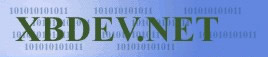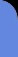Sunday July 12, 2020
 home | about | contact | Donations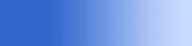Slice of Java. Its simple and its powerful...

No Walking Through Walls - Simple Collision Detection

by Ben Kenwright

I've been putting off writing this tutorial, as I think its one of the difficulties to explain - that is unless your really have a good feel for 3D vectors.  Hopefully with lots of coffee and a few diagrams....and of course my explanations, you'll have a grasp of how to do some simple collision detection.

Now there are a few fix's that you find you have to do to make it all work - and of course there's optimisation to look into...but what we'll do is get the ball rolling :)

 Here is the demo so you can see what we achieve:   Simply click on the Screenshot on the right to run the applet.  And you use the cursor keys or mouse cursor to move around.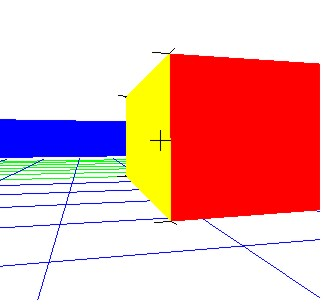How we'll merge the collision detection code into our applets is quiet easy.  Well create a main collision detection function called 'CollisionTest(..)' which will return 0 for no collision and 1 for a collision.  We'll then use this function to test for an intersection in our movement code...so when you press the up cursor key for example, before moving forwards...we'll pass the data to our CollisionTest(..) function and see if we can move to that new location....if its okay we move :)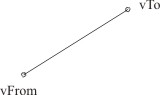int CollisionTest( Vector3 vFrom, Vector3 vTo ) {     ...     return 0; // No Collision }

So this is our collision detection function that we'll build up.  You could always rename it to IntersectionTest(..) if you prefer, as its common to check for collisions using intersections and use a collision response set of code separately.  But I think this way is the easiest.

Rather than pass the array of triangles, and the number of triangle to the function, which I  might change in a later date - so it makes our code more self contained.  I use reference to the two member variables:

 int m_NumTris;  // Number of triangle Triangle[] m_tri;  // Array of the triangles

Our 3D world...or simple maze in this demo, is made up of triangles.  Lots and lots of them.  So my first method of collision detection is to check a ray against each triangle.  Yup, its a bit brute force, but we can optimise it later.  And anyhow, we don't have that many triangles yet.  So for every triangle we will check if our line (vFrom->vTo) intersects with it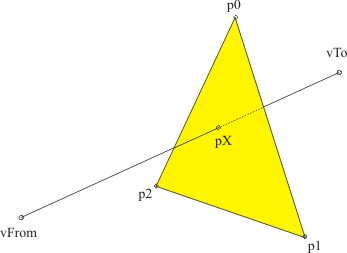I found it better to sum all the collisions together, rather than break away when we find the first one.  But thinknig about it now, we could probably optimise the code a bit by simply checking for the first collision and exiting from our 'TriangleLineCollision(..)' function and return 1.

The code is broken down into 3 main parts - checking for a collision on a plane with our line, then obtaining the collision point the plane....and finally, but probably the most confusing, is the sum of angles to all the points on our triangle edge from our point on the plane.  To determine if the point (pX) is actually inside or outside the plane.

 Line-Triangle Collision Testing int CollisionTest( Vector3 vFrom, Vector3 vTo )    {             int iCollisions = 0;             iCollisions += TriangleLineCollision(vFrom, vTo);             return iCollisions;      }// CollisionTest(..)        // Return 1 for a collision or 0 for no collision    int TriangleLineCollision( Vector3 vFrom, Vector3 vTo )    {             int iCollisions = 0;                           // Lets transform all of the triangles       for(int i=0; i 0.0f ) continue;               // -2- Get the point on the plane             Vector3 px = PointOnPlaneAlt( vFrom, vTo,                                         vTriNormal, p0 );               // -3- Is the point inside our outside our triangle             // I've expanded out the loop here, but we are checking to see             // if our point on the plane is inside the triangle - we use             // an old but common method - which is to add up all the angles to             // all the points on the edge of the triangle             // 2*PI => inside triangle             // else where outside the triangle             float angle = 0;                                           Vector3 vS, vT, vR;                                           Vector3 o1 = p0;             Vector3 o2 = p1;             Vector3 o3 = p2;                                           vS = Vector3.subtract(o1, px );             vT = Vector3.subtract(o2, px );             vS = Vector3.normalize( vS );             vT = Vector3.normalize( vT );             float cosAngle = Vector3.dot( vS, vT );             angle += Math.acos( cosAngle );                                           vS = Vector3.subtract(o2, px );             vT = Vector3.subtract(o3, px );             vS = Vector3.normalize( vS );             vT = Vector3.normalize( vT );             cosAngle = Vector3.dot( vS, vT );             angle += Math.acos( cosAngle );                                           vS = Vector3.subtract(o3, px );             vT = Vector3.subtract(o1, px );             vS = Vector3.normalize( vS );             vT = Vector3.normalize( vT );             cosAngle = Vector3.dot( vS, vT );             angle += Math.acos( cosAngle );                                           if( angle > (3.13f*2) )             {                iCollisions++;                m_tri[i].c = Color.orange;             }                           }// End for loop             return iCollisions;    }// End of TriangleLineCollision(..)

We can optimise our 'TriangleLineCollision(..)' detection function later on, as there are a few other more optimised versions of this around - which don't have to go through the process of summing up all the angles...which is the real drawback of this function.If our person is smart, he can move forwards and backwards at an angle to the wall, and he can escape!  Also we have a viewing area that would show part of the other side of the wall even if they arn't passed it.....so we need to keep the camera (e.g. first person view) a certain distance from the wall.

A simple fix to the above problem that I came up with, was to add a simple sphere-point collision detection....as I can give the starting and ending points a radius - and then make sure that spheres for our vFrom and vTo don't intersect any of the points that make up our triangle.

I then added a bit of a further tweak - I check that the actual line crosses the triangles plane before doing this check.Visitor: 9534626  { 209.237.238.175 } Copyright (c) 2002-2020 xbdev.net - All rights reserved. Designated tutorial and software are the property of their respective owners.